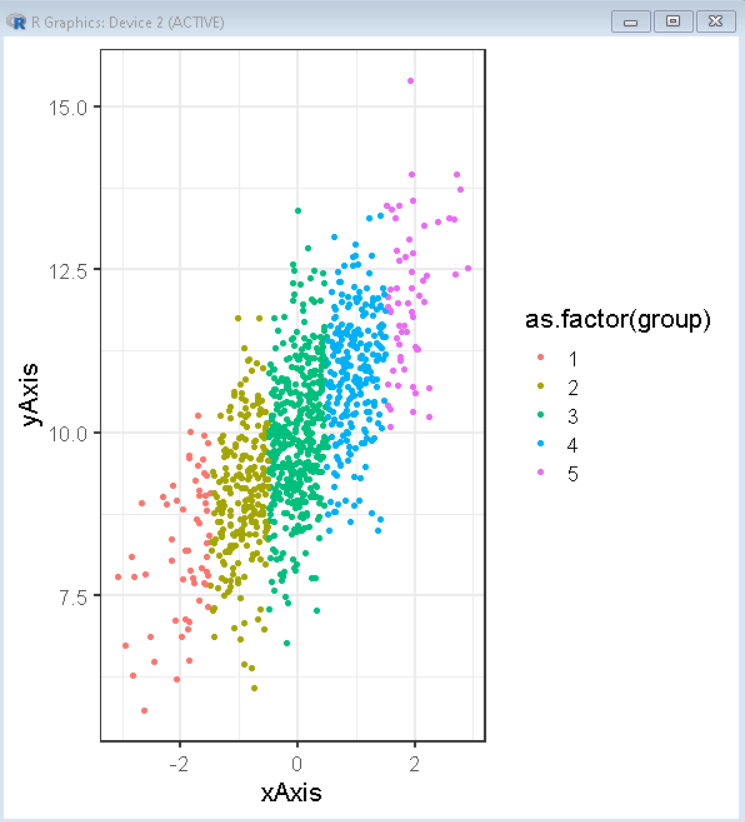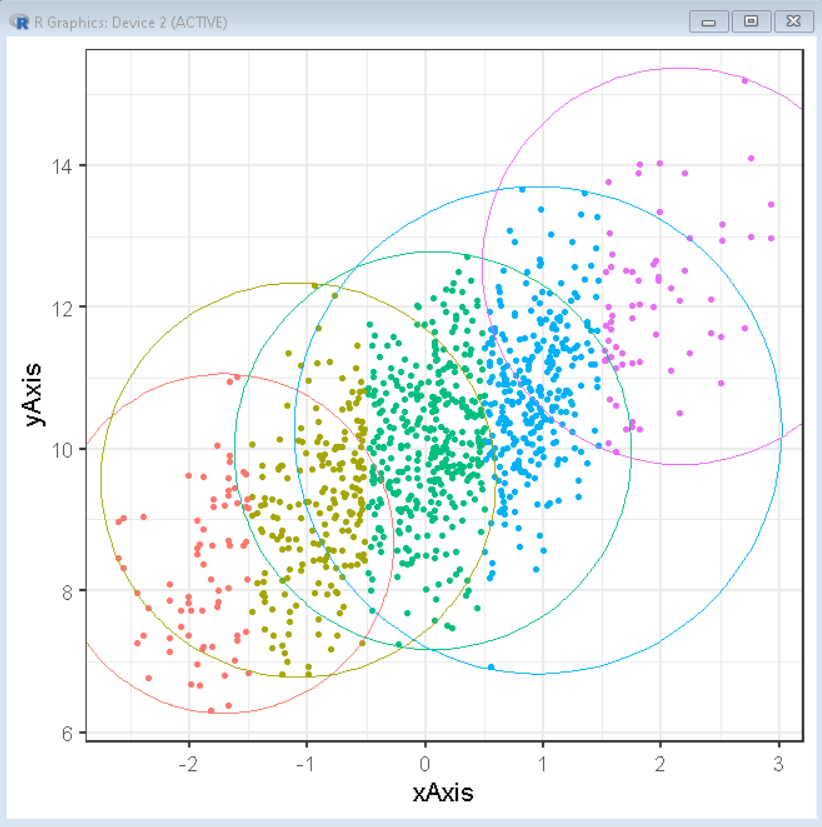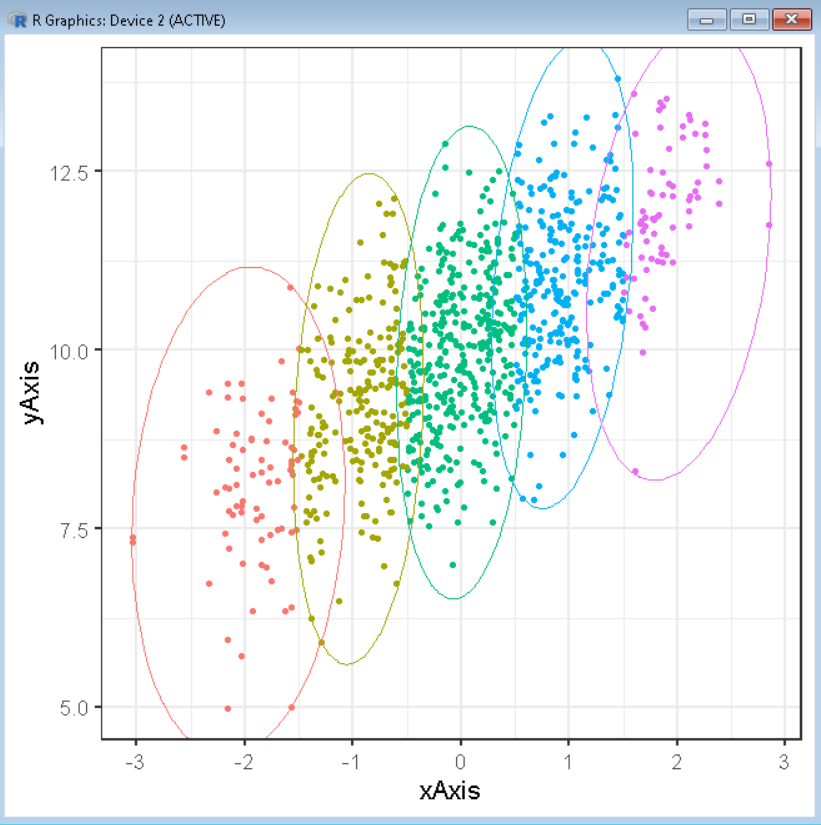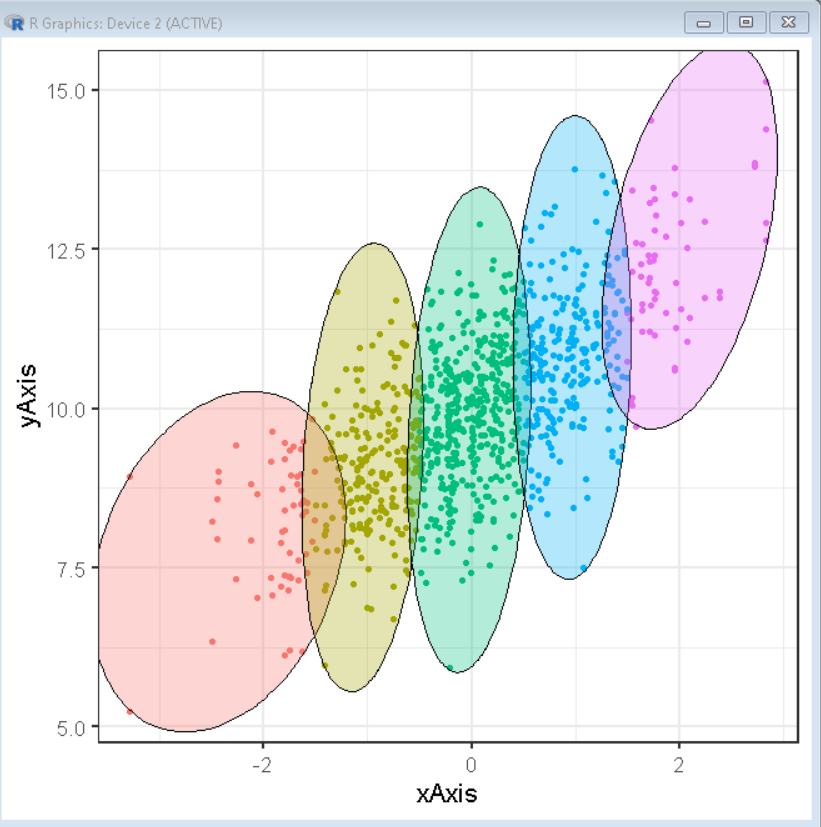# How To Annotate Clusters with Circle/Ellipse by a Variable in R ggplot2

• Last Updated : 24 Mar, 2022

In this article, we will discuss how to annotate Clusters with Circle/Ellipse by a categorical variable in the R Programming Language using the ggplot2 package.

To add a circle or ellipse around a cluster of data points, we use the geom_mark_circle() and geom_mark_ellipse() function of the ggforce package. This function automatically computes the circle/ellipse radius to draw around the cluster of points by categorical data.

First, we will plot the data in a scatter plot using the geom_point function of the ggplot2 package. We will use the color parameter of the aes() function to color the plot by a categorical variable group.

Syntax:

`ggplot(df, aes( x, y ) ) + geom_point( aes( color ))`

Arguments:

• df: determines the data frame to be used.
• x and y: determine the x-axis and y-axis variables respectively.
• color: determines the categorical variable for coloring the data point clusters.

Example:

Here, is a basic scatter plot made using the geom_point() function of the ggplot2 package. We have colored the plot by the categorical variable group.

## R

 `# load library tidyverse` `library``(tidyverse)`   `# set theme` `theme_set``(``theme_bw``(16))`   `# create x and y vector` `xAxis <- ``rnorm``(1000)                 ` `yAxis <- ``rnorm``(1000) + xAxis + 10    `   `# create groups in variable using conditional ` `# statements` `group <- ``rep``(1, 1000)              ` `group[xAxis > -1.5] <- 2` `group[xAxis > -0.5] <- 3` `group[xAxis > 0.5] <- 4` `group[xAxis > 1.5] <- 5`   `# create sample data frame` `sample_data <- ``data.frame``(xAxis, yAxis, group) `   `# create a scatter plot with points colored by` `# group` `ggplot``(sample_data, ``aes``(x = xAxis,` `             ``y = yAxis))+` `  ``geom_point``(``aes``(color = ``as.factor``(group)))`

Output:## Annotate circles around cluster:

To annotate a circle around a cluster of points by the group we use the geom_mark_circle() function of the ggforce package. To use this function we first install & import the ggforce package by using:

```install. packages('ggforce')
library(ggforce)```

Now, we will annotate the circle around a cluster of data points by using the geom_mark_circle() function.

Syntax:

ggplot(df, aes( x, y ) ) + geom_point( aes( color )) + geom_mark_circle( aes(color) )

Example:

Here, is a basic scatter plot with circles around a cluster of data points colored by a categorical variable group.

## R

 `# load library tidyverse` `library``(tidyverse)` `library``(ggforce)`   `# set theme` `theme_set``(``theme_bw``(16))`   `# create x and y vector` `xAxis <- ``rnorm``(500)                 ` `yAxis <- ``rnorm``(1000) + xAxis + 10    `   `# create groups in variable using conditional` `# statements` `group <- ``rep``(1, 500)              ` `group[xAxis > -1.5] <- 2` `group[xAxis > -0.5] <- 3` `group[xAxis > 0.5] <- 4` `group[xAxis > 1.5] <- 5`   `# create sample data frame` `sample_data <- ``data.frame``(xAxis, yAxis, group) `   `# create a scatter plot with points colored by group` `# circles are annotated using geom_mark_circle() function` `ggplot``(sample_data, ``aes``(x = xAxis,` `             ``y = yAxis))+` `  ``geom_point``(``aes``(color = ``as.factor``(group)))+` `  ``geom_mark_circle``(``aes``(color = ``as.factor``(group)), expand = ``unit``(0.5,``"mm"``))+` `  ``theme``(legend.position = ``"none"``)`

Output:## Annotate ellipses around cluster:

To annotate an ellipse around a cluster of points by the group we use the geom_mark_ellipse() function of the ggforce package. This function automatically computes the dimensions of the ellipse and overlays it on top of the scatter plot.

Syntax:

ggplot(df, aes( x, y ) ) + geom_point( aes( color )) + geom_mark_ellipse( aes(color) )

Example:

Here, is a basic scatter plot with ellipses around a cluster of data points colored by a categorical variable group.

## R

 `# load library tidyverse` `library``(tidyverse)` `library``(ggforce)`   `# set theme` `theme_set``(``theme_bw``(16))`   `# create x and y vector` `xAxis <- ``rnorm``(500)                 ` `yAxis <- ``rnorm``(1000) + xAxis + 10    `   `# create groups in variable using conditional` `# statements` `group <- ``rep``(1, 500)              ` `group[xAxis > -1.5] <- 2` `group[xAxis > -0.5] <- 3` `group[xAxis > 0.5] <- 4` `group[xAxis > 1.5] <- 5`   `# create sample data frame` `sample_data <- ``data.frame``(xAxis, yAxis, group) `   `# create a scatter plot with points colored by group` `# ellipses are annotated using geom_mark_ellipse() function` `ggplot``(sample_data, ``aes``(x = xAxis,` `             ``y = yAxis))+` `  ``geom_point``(``aes``(color = ``as.factor``(group)))+` `  ``geom_mark_ellipse``(``aes``(color = ``as.factor``(group)), expand = ``unit``(0.5,``"mm"``))+` `  ``theme``(legend.position = ``"none"``)`

Output:## Customizing the aesthetics

We can customize the aesthetics of the geom_mark_* function by using the color, fill, and alpha property of the aes() function.

Syntax:

ggplot(df, aes( x, y ) ) + geom_point( aes( color )) + geom_mark_ellipse( aes(color, fill, alpha) )

where,

• color: determines the color of the boundary of the circles or ellipses.
• fill: determines the background color of the circles or ellipses.
• alpha: determines the transparency of the circles or ellipses.

Example:

In this example, we will plot a  scatter plot overlayed by ellipses with a background colored by the group categorical variable.

## R

 `# load library tidyverse` `library``(tidyverse)` `library``(ggforce)`   `# set theme` `theme_set``(``theme_bw``(16))`   `# create x and y vector` `xAxis <- ``rnorm``(500)                 ` `yAxis <- ``rnorm``(1000) + xAxis + 10    `   `# create groups in variable using conditional` `# statements` `group <- ``rep``(1, 500)              ` `group[xAxis > -1.5] <- 2` `group[xAxis > -0.5] <- 3` `group[xAxis > 0.5] <- 4` `group[xAxis > 1.5] <- 5`   `# create sample data frame` `sample_data <- ``data.frame``(xAxis, yAxis, group) `   `# create a scatter plot with points colored by group` `# ellipses are annotated using geom_mark_ellipse() function` `ggplot``(sample_data, ``aes``(x = xAxis,` `             ``y = yAxis))+` `  ``geom_point``(``aes``(color = ``as.factor``(group)))+` `  ``geom_mark_ellipse``(``aes``(fill = ``as.factor``(group)), expand = ``unit``(0.5,``"mm"``))+` `  ``theme``(legend.position = ``"none"``)`

Output:My Personal Notes arrow_drop_up
Recommended Articles
Page :